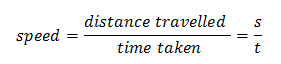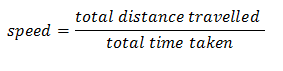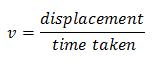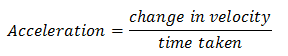# Motion

## 3. Speed

• Speed is defined as the total distance travelled by the object in the time interval during which the motion takes place. SI unit of speed is meter per second. So,where s is the distance travelled by the body and t is the time taken by the body to travel distance s.
• Speed of a body gives us the idea how slow or fast that body is moving.
• The ratio of total distance to total time taken by the body gives its average speed. The speed of a body at a given instant is its instantaneous speed.• A body is said to have constant or uniform speed if it travels equal distance in equal intervals of time.

## 4. Velocity

• The rate of change of displacement of a body with the passage of time is known as velocity of the body. Velocity of an object is measured in meter per second in SI units. So,• Velocity is nothing but the speed of an object moving in a definite direction.
• The velocity of an object can be uniform or variable. It can be changed by changing the object�s speed, direction of motion or both.
• So velocity of a body is a vector quantity involving both distance and displacement whereas speed of a body is a scalar quantity and it only has magnitude and does not have specific direction.
• Thus a body is said to be moving with uniform velocity if it covers equal distances in equal intervals of time in a specified direction.
• A body is said to be moving with non uniform velocity if it covers unequal distances in equal intervals of time and vice-versa in a specified direction or if it changes the direction of motion.
• The velocity of a body can be changed in two ways first by changing the speed of the body and second by changing the direction of motion of the body by keeping the speed constant. Also both speed and direction of the body can be varied in order to change the velocity of the body.
• When velocity of the object changes at a uniform rate, then average velocity is given by the arithmetic mean of initial velocity and final velocity for a given period of time. That is,Where u is the initial velocity of the object and v is the final velocity of the object.

## 5. acceleration

• Acceleration is a measure of the change in the velocity of an object per unit time and mathematically it is given as• If the velocity of an object changes from an initial value u to the final value v in time t, the acceleration a is given by,
$a = \frac{{v - u}}{t}$
and this kind of motion is called accelerated motion.
• A body has uniform acceleration if it travels in a straight line and its velocity increases by equal amount in equal intervals of time for example freely falling bodies, motion of ball rolling down the inclined plane etc.
• A body has non uniform acceleration if its velocity increases or decreases by unequal amount in equal intervals of time.
• If acceleration is in the direction of the velocity then it is positive acceleration and if it is in the direction opposite to the direction of velocity then it is negative and the negative acceleration is termed retardation.
• SI unit of acceleration is ms-2.

Fill in the Blank Questions
1)  __________________ is defined as the total distance travelled by the body in the time interval during which the motion takes place.
2) __________________ unit of speed is m/s
3) The ratio of total distance to total __________________ taken by the body gives its average speed.
4) The rate of change of displacement of a object with the passage of time is known as __________________ of the object.
5) velocity of a body is a vector quantity involving both distance and __________________ whereas speed of a body is a scalar quantity and it only has magnitude  and does not have specific direction.
6) A body is said to be moving with uniform velocity if it covers equal distances in equal intervals of time in a specified __________________.
7) A object  is said to be moving with non uniform velocity if it covers unequal distances in equal intervals of time and vice-versa in a specified direction or if it
changes the direction of __________________.
8) If acceleration is in the __________________ of the velocity then it is positive acceleration and if it is in the __________________ opposite to the ___________
_______ of velocity then it is negative and the negative acceleration is termed retardation.

True and False Statement
1) Acceleration is a measure of the change in the speed of an object per unit time
2)  A body has Non Uniform acceleration if its velocity increases or decreases by unequal amount in equal intervals of time.
3) When velocity of the object changes at a uniform rate, then average veloicty is given by the arithmetic mean of initial velocity and final velocity for a given period of time.
4) The velocity of a body can be changed in two ways first by changing the speed of the object  and second by changing the direction of motion of the body by keeping the speed constant.
5) The odometer in the car measures the velocity of the car
6) A object is said to have constant or uniform velocity  if it travels equal distance in equal intervals of time.
7) A body has uniform acceleration if it travels in a straight line and its velocity increases by equal amount in equal intervals of time

Reference Books for class 9 science

Given below are the links of some of the reference books for class 9 science.

These books by S.Chand Publications are detailed in their content and are must have books for class 9 students. Some other books that might be worth considering are
1. Oswaal CBSE Question Bank Class 9 Science Chapterwise & Topicwise We all know importance of practicing what we have studied. This book is perfect for practicing what you have learned and studied in the subject.
2. Pearson Foundation Series (IIT-JEE/NEET) Physics, Chemistry, Maths & Biology for Class 9 (Main Books) | PCMB Combo These Foundation books would be helpful for students who want to prepare for JEE/NEET exams. Only buy them if you are up for some challenge and have time to study extra topics. These might be tough for you and you might need extra help in studying these books.
3. Foundation Science Physics for Class - 9 by H.C. Verma This is one of my favorite Physics book for class 9. Most of the book is within the limits of CBSE syllabus. It might overwhelm you with its language but I feel if you can understand the content is authentic with plenty of problems to solve.

You can use above books for extra knowledge and practicing different questions.

Note to our visitors :-

Thanks for visiting our website.